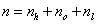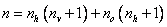Home News About Me Ph. D. Thesis Abstract Table of Contents 1. Introduction 2. Theory  Fundamentals of the Multivariate Data Analysis 2.1. Overview of the Multivariate Quantitative Data Analysis 2.2. Experimental Design 2.3. Data Preprocessing 2.4. Data Splitting and Validation 2.5. Calibration of Linear Relationships 2.6. Calibration of Nonlinear Relationships 2.7. Neural Networks  Universal Calibration Tools 2.8. Too Much Information Deteriorates Calibration 2.8.1. Overfitting, Underfitting and Model Complexity 2.8.2. Neural Networks and the Complexity Problem 2.8.3. Brute Force Variable Selection 2.8.4. Variable Selection by Stepwise Algorithms 2.8.5. Variable Selection by Genetic Algorithms 2.8.6. Variable Selection by Simulated Annealing 2.8.7. Variable Compression by Principal Component Analysis 2.8.8. Topology Optimization by Pruning Algorithms 2.8.9. Topology Optimization by Genetic Algorithms 2.8.10. Topology Optimization by Growing Neural Network Algorithms 2.9. Measures of Error and Validation 3. Theory  Quantification of the Refrigerants R22 and R134a: Part I 4. Experiments, Setups and Data Sets 5. Results  Kinetic Measurements 6. Results  Multivariate Calibrations 7. Results  Genetic Algorithm Framework 8. Results  Growing Neural Network Framework 9. Results  All Data Sets 10. Results  Various Aspects of the Frameworks and Measurements 11. Summary and Outlook 12. References 13. Acknowledgements Publications Research Tutorials Downloads and Links Contact Search Site Map Print this Page### 2.8.2.   Neural Networks and the Complexity Problem

The complexity of neural networks can be mainly reduced to the number of adjustable parameters, namely the number of weights and the number of biases. Although the number of hidden layers also seems to influence the complexity of the neural networks on the first sight, the dimensionality of the optimization hyperspace and with it the complexity of the model is only defined by the number of adjustable parameters while the number of hidden layers influences only the direction of the optimization walk in the hyperspace. Consequently, the number of parameters can be ascribed to the sum of links, hidden neurons and output neurons. The number of adjustable parameters n can be calculated as:(12)

Thereby nl is the number of all links in the network, nh is the number of hidden neurons and no is the number of output neurons. For uniform fully connected networks, the number of links can be replaced:(13)

with nv as the number of input variables (input neurons). Consequently, several strategies are possible for optimizing the model complexity and thus the neural networks :

1. Optimizing the number of input variables nv by a variable selection strategy.
2. Optimizing the number of hidden layers and the number of neurons in the hidden layers nh.
3. Building networks with an arbitrary non-uniform structure optimized for the specific problem and thus directly optimizing the number of links nl and hidden neurons nh.

The first strategy is known as feature selection or variable selection  and has been proposed and applied in numerous publications. Thereby an optimal subset of variables is selected, avoiding an overfitting and the inclusion of too noisy and redundant variables, which could hide meaningful variables ,. Additionally, the danger of chance correlation, which is further discussed in chapter 7, decreases with fewer variables . Many variable selection strategies have been proposed and compared  such as the two stepwise algorithms forward addition and backward elimination -, such as orthogonal descriptors and successive projection algorithms for linear calibration models , and such as simulated annealing - and genetic algorithms ,-, which contain random search steps. Especially the last two approaches have been demonstrated to be superior in the case of many variables -,. A compression of the input variables by the use of a principal component analysis can also help to reduce the number of parameters -. The most important approaches are discussed in detail in the sections 2.8.3 to 2.8.10.

The second strategy optimizes the inner topology of the neural networks by varying the number of hidden layers and number of neurons in the hidden layers, whereby the networks are fully connected. In most publications dealing with neural networks, this task is performed by a trial and error procedure and relies on the intuition of the user. Yet, there are two non-manual approaches for finding an optimal inner topology of neural networks. The first approach is based on the use of genetic algorithms for finding the optimal number of hidden layers and hidden neurons whereby the number of neurons of each layer is directly coded into the genetic string . This approach is usually combined with a variable selection based on genetic algorithms ,. The second approach is based on neural networks with growing hidden layers. Thereby full-connected hidden neurons are added to a single hidden layer ,- or to a prespecified location (known as cascade correlation architecture ) until the error of prediction does not improve any more. All approaches based on the second strategy are faced by 2 major drawbacks: Only fully connected neurons are added to the network resulting in a simultaneous addition of many parameters per addition step and the place where to add the neurons is not optimized by any of the algorithms.

The third strategy, which is also known as topology optimization, should be superior to the first two strategies since both, the number of input variables and the number and location of the hidden neurons are simultaneously optimized resulting in a synergetic effect. Additionally, the presence of each single possible link and with it each single parameter is decided on when building non-uniform networks. Thus, the number of adjustable parameters is effectively reduced, allowing the iterative calibration procedure of neural networks to find a better solution  than fully connected networks. Three general approaches can be found in literature following this strategy. The first approach is based on a structure generation and evaluation by the use of genetic algorithms -. In the second approach unimportant links of a big network are removed by so-called pruning techniques ,,-. The third approach is based on growing non-uniform neural networks proposed by Vinod et al. . In the next sections, the different methods for selecting and compressing variables are discussed and then the three approaches for the optimization of the network topology are looked at in more detail.

 Page 49 © Frank Dieterle, 03.03.2019